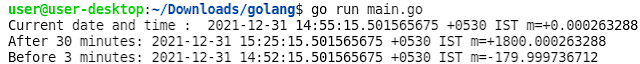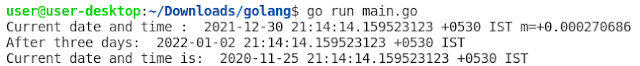## Posts

Showing posts from December, 2021

### How to add/subtract Hour, Minute, Second, Millisecond, Microsecond and Nanosecond to a date in Go LanguageHello everyone, In this tutorial, we will learn h ow to add/subtract Hour, Minute, Second, Millisecond, Microsecond and Nanosecond to date in Go Language. The time package in Go language provides Add() function which  is used to add/subtract a duration to time t. Since duration can be represented in hours, minutes, seconds, milliseconds, microseconds and nanoseconds, The signature of the Add method is: func (t Time) Add(d Duration) Time Example 1: Go Program to add/subtract minutes. package main import ( "fmt" "time" ) func main() { //Current date and time dt1 := time.Now() fmt.Println( "Current date and time : " , dt1) //Adding 30 minutes dt2 := dt1.Add( 30 * time.Minute) fmt.Println( "After 30 minutes:" , dt2) //Subtract 3 minutes dt3 := dt1.Add(- 3 * time.Minute) fmt.Println( "Before 3 minutes:" , dt3) } Output: Example 2: Go Program to add/subtract seconds. package main import (

### How to add/subtract days, months or years to a date in Go LanguageHello everyone, In this tutorial, we will learn h ow to add/subtract days, months or years to a date in Go Language. The time package in Go language provides AddDate() function which is used to add/subtract years, months and days to time t.  The signature of the AddDate method is: func (t Time) AddDate(years int , months int , days int ) Time Example 1: Go Program to add/subtract days. package main import ( "fmt" "time" ) func main() { //Current date and time dt1 := time.Now() fmt.Println( "Current date and time : " , dt1) //Adding 3 days dt2 := dt1.AddDate( 0 , 0 , 3 ) fmt.Println( "After three days: " , dt2) //Subtract 400 days dt3 := dt1.AddDate( 0 , 0 , - 400 ) fmt.Println( "Before 400 days: " , dt3) } Output: Example 2: Go Program to add/subtract months. package main import ( "fmt" "time" ) func main() { //Current date and time dt1 := time.Now()

### How to iterate over all the characters of the string in Go Language

In Go Language, a string is a slice of bytes. The bytes of the strings can be defined in the Unicode text using UTF-8 encoding. UTF-8 is based on 8-bit code units. Each character is encoded as 1 to 4 bytes. The first 128 Unicode code points are encoded as 1 byte in UTF-8. For Example, package main import "fmt" func main() { text := "aπ" fmt.Println(len(text)) } Output: 3 Here the length of the String is 3 because, ‘a’ takes one byte as per UTF-8  ‘π’ takes two bytes as per UTF-8 So we cannot use for loop to iterate over all the characters of the string because  it will iterate over bytes and not characters. This means  it will iterate three times and the print value corresponding to a byte at that index. How to iterate over all the characters of the string in Go Language? Iterate over a string by runes  Iterate over a string by for-range loop Iterate over a string by runes Go allows us to easily convert a string to a slice of runes and then iterate over t